# Test Prep Plan - Take a practice test

Take this practice test to check your existing knowledge of the course material. We'll review your answers and create a Test Prep Plan for you based on your results.
How Test Prep Plans work
1
2Based on your results, we'll create a customized Test Prep Plan just for you!
3Study smarter
Study more effectively: skip concepts you already know and focus on what you still need to learn.

# ASVAB Math: Properties of Exponents Chapter Exam

Exam Instructions:

Choose your answers to the questions and click 'Next' to see the next set of questions. You can skip questions if you would like and come back to them later with the yellow "Go To First Skipped Question" button. When you have completed the practice exam, a green submit button will appear. Click it to see your results. Good luck!

### Page 1

#### Question 1 1. Which of the following is equivalent to the expression below?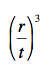#### Question 2 2. Simplify the expression below using positive exponents only.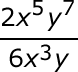#### Question 3 3. Simplify the expression below.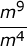#### Question 4 4. Which of the following is equivalent to the following expression?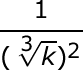#### Question 5 5. Simplify using only positive exponents.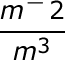### Page 2

#### Question 6 6. Which of the following is equivalent to the following expression?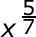#### Question 7 7. Which of the following is equivalent to the expression below?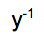#### Question 8 8. Simplify using ONLY positive exponents.#### Question 9 9. Which of the following radical expressions is equal to this exponential expression?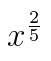#### Question 10 10. Which of the following would be true regarding the following exponential expression?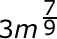### Page 3

#### Question 11 11. Simplify this expression.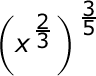#### Question 12 12. Simplify using ONLY positive exponents.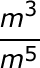#### Question 13 13. Simplify the following expression using positive exponents only.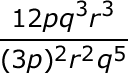#### Question 14 14. Which of the following exponential expressions is equal to this radical expression?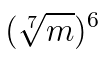#### Question 15 15. Simplify the expression below using only positive exponents.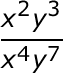### Page 4

#### Question 16 16. Which of the following is equivalent to the expression below?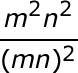#### Question 18 18. Simplify the following expression.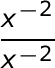#### Question 19 19. Simplify the following expression.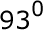#### Question 20 20. Simplify this expression so that all the exponents are positive.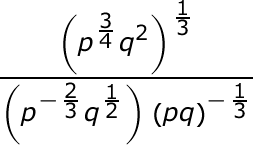### Page 5

#### Question 21 21. Simplify the expression and make sure all the exponents are positive in the answer.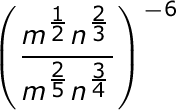#### Question 22 22. Simplify the following expression so that all the exponents are positive.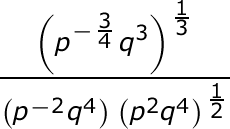#### Question 23 23. Simplify the following expression using positive exponents.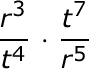#### Question 24 24. Which of the following is equivalent to the expression below?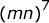#### Question 25 25. Simplify the expression: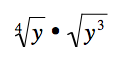#### ASVAB Math: Properties of Exponents Chapter Exam Instructions

Choose your answers to the questions and click 'Next' to see the next set of questions. You can skip questions if you would like and come back to them later with the yellow "Go To First Skipped Question" button. When you have completed the practice exam, a green submit button will appear. Click it to see your results. Good luck!

Support